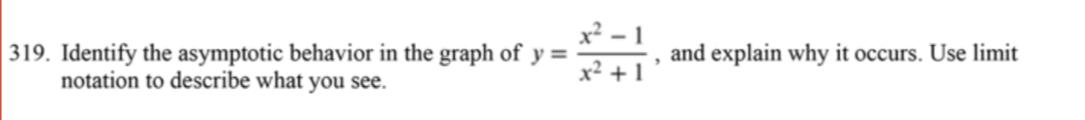Home / Expert Answers / Other Math / 19-identify-the-asymptotic-behavior-in-the-graph-of-y-x2-1x21-and-explain-why-it-occurs-u-pa194

# (Solved): 19. Identify the asymptotic behavior in the graph of y=x2+1x21, and explain why it occurs. U ...19. Identify the asymptotic behavior in the graph of , and explain why it occurs. Use limit notation to describe what you see.

We have an Answer from Expert

VERTICAL ASYMPTOTES
The line x=L is a vertical asymptote of the function   , if the limit of the function (one-sided) at this point is infinite.
In other words, it means that possible points are points where the denominator equals 0 or doesn't exist.
So, find the points where the denominator equals 0 and check them.
As can be seen, there are no such points, so this function doesn't have vertical asymptotes.

We have an Answer from Expert